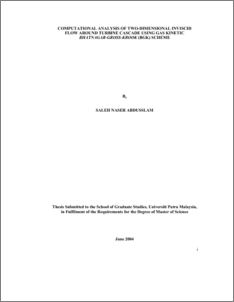# Computational Analysis of Two-Dimensional Inviscid Flow Around Turbine Cascade Using Gas Kinetic Bhatnagar-Gross-Krook (BGK) Scheme

## Citation

Abdusslam, Saleh Naser (2004) Computational Analysis of Two-Dimensional Inviscid Flow Around Turbine Cascade Using Gas Kinetic Bhatnagar-Gross-Krook (BGK) Scheme. Masters thesis, Universiti Putra Malaysia.

## Abstract

Fluid flows within turbomachinery tend to be extremely complex. Understanding such flows is crucial to improve current turbomachinery designs, and the computational approach can be used to great advantage in this regard. This study presents a gas kinetic Bhatnagar-Gross-Krook (BGK) scheme to calculate the flow within a turbine cascade. The two dimensional Euler equations with a numerical method based on the gas kinetic Bhatnagar-Gross-Krook (BGK) scheme was developed to simulate the flow around a turbine cascade configuration. The grids around the select model are efficiently generated using a multi-zone structure technique.Computed results for the even studied cases were compared with experimental data in terms of Mach number distribution. Comparisons of these results with experimental data were made wherever possible and the agreement between the two is found to be good. In addition the gas kinetic Bhatnagar-Gross-Krook (BGK) scheme as the numerical procedure has been tested for its accuracy in solving the two-dimensional compressible inviscid flow fields. Analyzing the results in regular shock reflection revealed that the gas kinetic Bhatnagar-Gross-Krook (BGK) scheme is able to produce numerical results that are comparable, robust, and accurate as Roe’s scheme. In addition, the gas kinetic Bhatnagar-Gross-Krook (BGK) scheme converges faster than Roe's scheme. For a given 1000 time iterations, the BGK scheme converges at 887 time iterations while the Roe's scheme converges at 906 time iterations. Therefore, the BGK scheme proved to be more efficient than Roe’s scheme.Preview
PDF
549512_FK_2004_76.pdfView Item